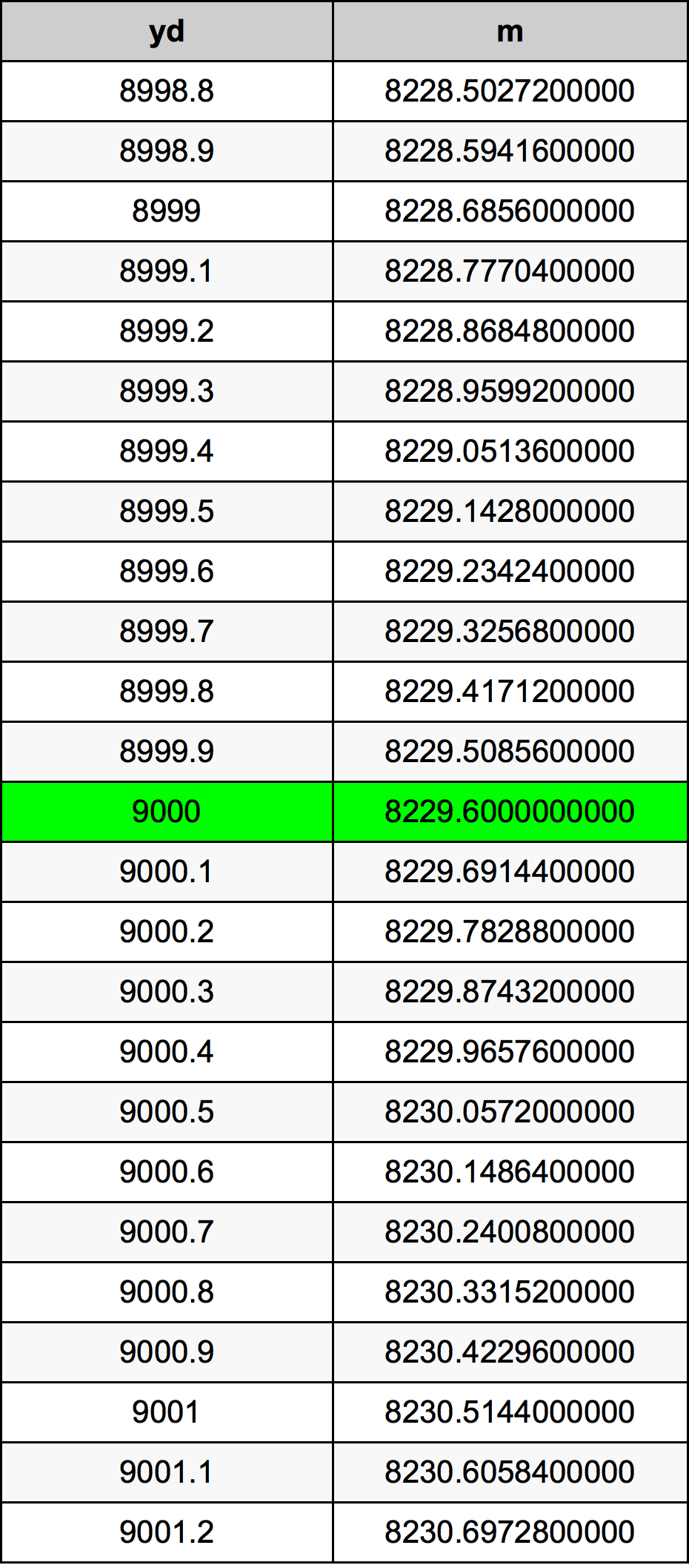Yards To Meters

# 9000 yd to m9000 Yards to Meters

yd
=
m

## How to convert 9000 yards to meters?

 9000 yd * 0.9144 m = 8229.6 m 1 yd
A common question is How many yard in 9000 meter? And the answer is 9842.51968504 yd in 9000 m. Likewise the question how many meter in 9000 yard has the answer of 8229.6 m in 9000 yd.

## How much are 9000 yards in meters?

9000 yards equal 8229.6 meters (9000yd = 8229.6m). Converting 9000 yd to m is easy. Simply use our calculator above, or apply the formula to change the length 9000 yd to m.

## Convert 9000 yd to common lengths

UnitUnit of length
Nanometer8.2296e+12 nm
Micrometer8229600000.0 µm
Millimeter8229600.0 mm
Centimeter822960.0 cm
Inch324000.0 in
Foot27000.0 ft
Yard9000.0 yd
Meter8229.6 m
Kilometer8.2296 km
Mile5.1136363636 mi
Nautical mile4.4436285097 nmi

## What is 9000 yards in m?

To convert 9000 yd to m multiply the length in yards by 0.9144. The 9000 yd in m formula is [m] = 9000 * 0.9144. Thus, for 9000 yards in meter we get 8229.6 m.

## 9000 Yard Conversion Table## Alternative spelling

9000 Yards to m, 9000 Yards in m, 9000 Yard to m, 9000 Yard in m, 9000 yd to Meters, 9000 yd in Meters, 9000 yd to m, 9000 yd in m, 9000 yd to Meter, 9000 yd in Meter, 9000 Yard to Meter, 9000 Yard in Meter, 9000 Yards to Meter, 9000 Yards in Meter